Stieltjes polynomials

A system of polynomials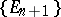which satisfy the orthogonality conditionwhere the weight functionsatisfies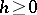,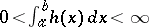, with finite moments.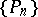is the system of orthogonal polynomials associated with. The degree ofis equal to its index. The orthogonality conditions defineup to a multiplicative constant, but the conditions forgiven above are not sufficient forto have real zeros in. However, several special cases and classes of weight functionsare known for which the zeros of the corresponding Stieltjes polynomials do not only have this property, but also interlace with the zeros of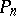. A simple example is,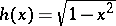,, the Chebyshev polynomial of the second kind (cf. Chebyshev polynomials), where, the Chebyshev polynomial of the first kind. For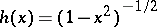,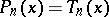and. A generalization are the Bernstein–Szegö weight functionswhereis a polynomial of degreethat is positive in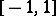[a6], [a7]. Weight functions for which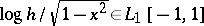,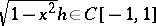andforare another class for which the above properties are known to hold asymptotically under certain additional conditions on[a8].

The classical case originally considered by Th.J. Stieltjes is the Legendre weight function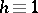,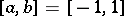. For this case G. Szegö [a9] proved that all zeros are real, belong to the open intervaland interlace with the zeros of the Legendre polynomials. Szegö extended his proof to the ultraspherical, or Gegenbauer, weight function,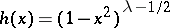,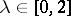,, cf. also Gegenbauer polynomials; Ultraspherical polynomials. For the more general Jacobi weight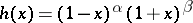, results of existence and non-existence can be found in [a4]. Comparatively little is known for unbounded intervals. Numerical results reported in [a5] show that complex zeros arise for the Laguerre weight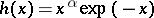,,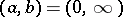and the Hermite weight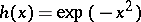,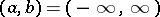.

An important fact for the analysis of the Stieltjes polynomials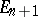is their close connection with the functions of the second kind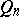associated withand. Stieltjes [a1] proved thatis precisely the polynomial part of the Laurent expansion of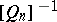. Szegö's work in [a9] and subsequent investigations are based on this connection.

Several asymptotic representations are available. A simple formula for the Legendre weight function isuniformly for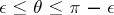, see [a2]. Inequalities for Stieltjes polynomials in the casecan be found in [a3].

The zeros of Stieltjes polynomials are used for quadrature and for interpolation. In particular, the often-used Gauss–Kronrod quadrature formulas (cf. Gauss–Kronrod quadrature formula) are based on the union of the zeros ofandand enable an efficient estimation for the Gauss quadrature formula based on the zeros of. This idea has been carried over to extended interpolation processes (cf. Extended interpolation process). For, adding the zeros ofimproves the interpolation process based on the zeros ofto an optimal-order interpolation process [a3] (see also Extended interpolation process).